# Chebyshev pseudo-spectral method

(diff) ← Older revision | Latest revision (diff) | Newer revision → (diff)

A type of trigonometric pseudo-spectral method (cf. Trigonometric pseudo-spectral methods). See also Fourier pseudo-spectral method.

A Chebyshev polynomial is defined as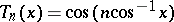(cf. also Chebyshev polynomials). If, the resulting Chebyshev function is truly anth order polynomial in, but it is also a cosine function with a change of variable. Thus, a finite Chebyshev series expansion is related to a discrete cosine transform.

The Chebyshev pseudo-spectral method is the most logical choice of pseudo-spectral methods for problems with non-periodic boundary conditions. This comes from the particularly nice characteristics of the Chebyshev interpolating polynomials (cf. also Chebyshev polynomials).

Of all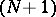st degree polynomials, with leading coefficient,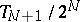has the smallest maximum on the interval. Thus, in Lagrangian interpolation (see also Lagrange interpolation formula), if the interpolation points are taken to be the zeros of this polynomial, the error is minimized. A related and possibly more useful set of interpolation points are the extrema of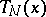: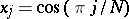,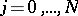, called the Gauss–Lobatto points.

The trigonometric interpolation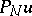of the functionat the Gauss–Lobatto points is, where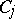is the Cardinal function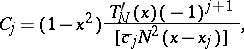with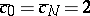,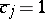otherwise. Rearranging, the interpolation polynomial becomes a finite Chebyshev series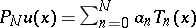, where the Chebyshev coefficients are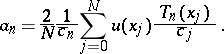Suppose the equationis to be solved, whereis a differential operator,is a given function andis an unknown function. In the Chebyshev pseudo-spectral method, the solutionis approximated by a Chebyshev interpolating polynomial. In the Lagrangian polynomial or "grid-point representation" , the problem can be written as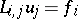, where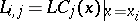. The form of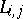can be found through differentiation of the Cardinal function:,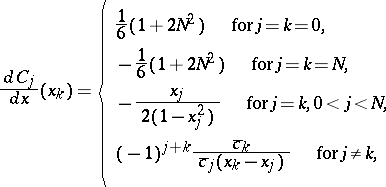and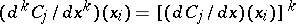. Note that derivatives requireoperations.

Another way to find an expression for the derivative is to differentiate the Chebyshev series, which means differentiating the Chebyshev polynomials and using the recurrence relation for derivatives of Chebyshev polynomials,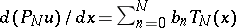, where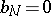,,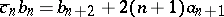for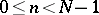. Thus, in coefficient representation, the derivative can be evaluated in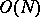operations while non-linear terms or multiplication by non-constant coefficients require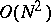. The fast discrete cosine transform can be used to switch between spectral and grid point representations.

How to Cite This Entry:
Chebyshev pseudo-spectral method. Encyclopedia of Mathematics. URL: http://encyclopediaofmath.org/index.php?title=Chebyshev_pseudo-spectral_method&oldid=14983
This article was adapted from an original article by Richard B. Pelz (originator), which appeared in Encyclopedia of Mathematics - ISBN 1402006098. See original article